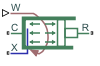# Rotating Cylinder Force (IL)

Centripetal pressure gradient for components in an isothermal liquid system

Since R2020a

•Libraries:
Simscape / Fluids / Isothermal Liquid / Actuators / Auxiliary Components

## Description

The Rotating Cylinder Force (IL) block models the axial force due to a centripetal pressure gradient in a rotating shaft about its symmetry axis, which is found in applications such as friction clutches, square-jaw positive clutches, dog clutches, and brakes. Connect this block in parallel to a Single-Acting Actuator (IL) or a Translational Mechanical Converter (IL) block to model a rotating actuator. The fluid density is recorded at the isothermal liquid port X. The angular velocity is received as a physical signal at port W. Port R is associated with the piston velocity, relative to the case at port C.

The angular velocity of the shaft is assumed to be constant or nearly-constant, and therefore the fluid is considered to be in rigid-body rotation.

The centripetal force is due solely to the fluid angular momentum induced by shaft rotation. Removing the contribution of the static pressure in the fluid channel, the contribution of the rotation of the shaft to the axial force is:

`${F}_{rotational}=\frac{\rho \pi {W}^{2}}{4}\left({r}_{o}^{4}-{r}_{i}^{4}-2{r}_{p}^{2}\left({r}_{o}^{2}-{r}_{i}^{2}\right)\right),$`

where:

• ρ is the fluid density at port X.

• W is the angular velocity of the rotating pipe, received as a physical signal at port W.

• ro is the Piston outer radius.

• ri is the Piston inner radius.

• rp is the Fluid entry radius.

## Ports

### Conserving

expand all

Liquid port associated with fluid density. There is no mass flow through port X. Connect port X to port A of the Single-Acting Actuator (IL) or Translational Mechanical Converter (IL) blocks.

Mechanical translational conserving port associated with the cylinder casing reference velocity and force.

Mechanical translational conserving port associated with the piston velocity and force induced by the pressure differential.

### Input

expand all

Angular velocity in rad/s, specified as a physical signal.

## Parameters

expand all

Radius of the fluid opening in the cylinder casing, measured from the rotational axis. This value must be greater than or equal to the Piston inner radius and less than or equal to the Piston outer radius.

Piston displacement direction of the connected actuator block. ```Pressure at A causes positive displacement of R relative to C``` corresponds to positive displacement, or actuator shaft extension, when RC is positive. ```Pressure at A causes negative displacement of R relative to C``` corresponds to negative displacement, or actuator shaft retraction, when RC is positive.

## Version History

Introduced in R2020a